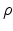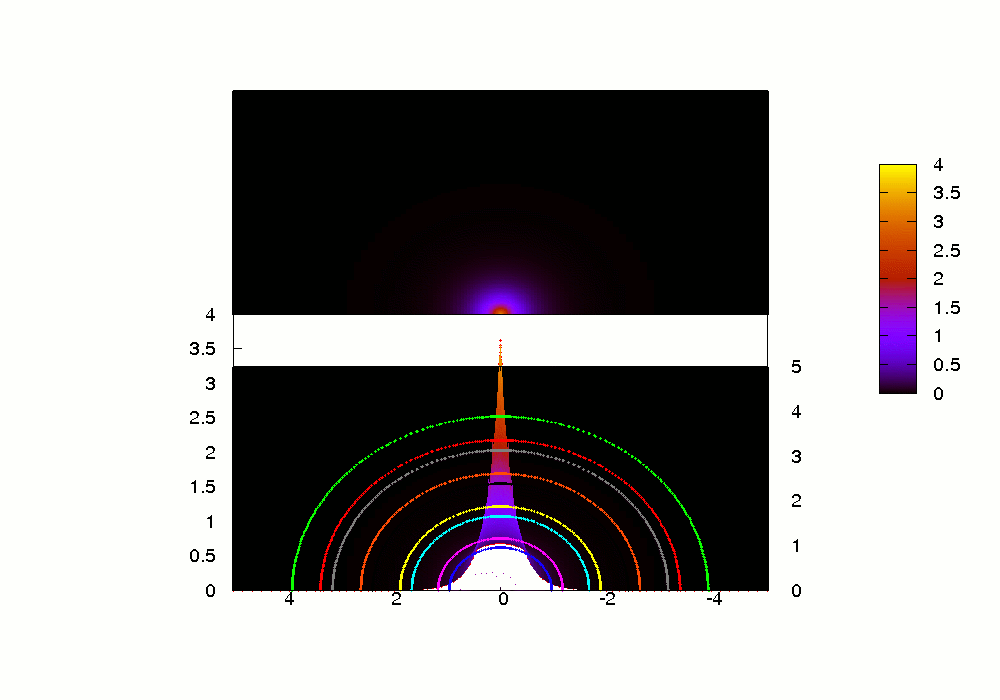Next: Resources Up: Density functional theory Previous: Using QFDFT to obtain

### Using QFDFT to study electron-density-based dynamics of select systems under intense laser field

In the earlier subsection, we had tested the efficacy of a single generalized non-linear Schrodinger equation in obtaining the ground state energies of the full spectrum of noble gas atoms in an appropriately scaled cylindrical coordinate system. We can make use of the same equation and the algorithm to study electron -density-dependent- phenomena in the attosecond domain in a helium atom under an intense laser field in scaled cylindrical coordinates. The close agreement (in most cases) of the results to those already in the literature validates the use of the above equation and the algorithm to the study of electron-density- based- dynamics of similar time-dependent systems in three dimensions. The computational simplicity involved makes the approach all the more attractive.

In the box below are presented a few pictures which show how the electron density oscillates with the same frequency of the external periodic perturbation provided by the intense laser field which is polarized along the z axis. The two-dimensional grids used in the plots pertain to the z-axis (horizontal) and-axis (vertical) in a cylindrical coordinate system. The polarization of the electric field along the z-axis ensures the cylindrical symmetry of the system (i.e. the effect of the azimuthal angle on the dynamics is averaged out). Both surface and contour plots have been provided. These plots have been obtained through the numerical solution of the Generalized nonlinear Schrodinger equation (GNLSE) which we introduced ourselves earlier. The plots have been generated using the gnuplot and pm3d software packages available under gnu public license.

Use the buttons at the bottom of the box to move from one picture to the other or view an animation.

 Electron density dynamics in an intense laser fieldThe surface plot + contour plot of the electron density when the intensity of the electric field is close to zero The surface plot + contour plot of the electron density when the electric field is maximum The surface + contour plot of the electron density when the intensity of the electric field returns to zero The surface plot + contour plot of the electron density when the electric field is minimum The surface + contour plot of the electron density when the intensity of the electric field returns to zero again

The animation is very intuitive in itself. It gives an idea how the electron density pulsates about its equilibrium zero-field profile. You might wonder what is the utility of following the temporal behaviour of the electron density. The answer lies in the fact that all time dependent phenomena can, in principle be understood through the study of the electron density.

The behaviour of many-electron systems under the influence of intense laser fields has been a very actively pursued research topic for the past few decades. The advent of very intense short laser pulses in the femtosecond range has made possible the study of many novel phenomena like single and multiple ionization as well as high harmonic generation. Of late, it has been possible to generate XUV pulses of extremely short (attosecond) duration by mixing high harmonics having equal phases. These pulses have the potential of probing electronic processes involving attosecond temporal resolution thus opening new vistas in research in atomic, molecular and optical physics.

As you might have already guessed, studies and experiments as above can be understood much simplistically and accurately too by following the dynamics of the electron density.Next: Resources Up: Density functional theory Previous: Using QFDFT to obtain
Abhijit Poddar
2007-09-27